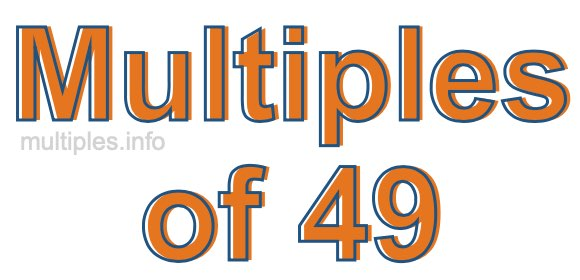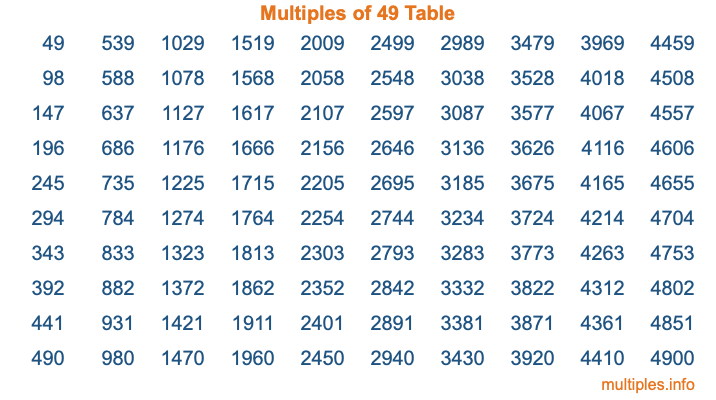Multiples of 49Welcome to the Multiples of 49 page. Here we will first teach you everything you will ever need to know about the multiples of 49, and then give you a study guide summary of everything we taught you to make sure you remember it all. Use this page to look up facts and learn information about the multiples of 49. This page will make you a multiples of forty-nine expert!

Definition of Multiples of 49
Multiples of 49 are all the numbers that when divided by 49 equal an integer. Each of the multiples of 49 are called a multiple. A multiple of 49 is created by multiplying 49 by an integer.

Therefore, to create a list of multiples of 49, you start with 1 multiplied by 49, then 2 multiplied by 49, then 3 multiplied by 49, and so on for as long as you want. Thus, the list of the first five multiples of 49 is 49, 98, 147, 196, and 245. To see a larger list of multiples of 49, see the printable image of Multiples of 49 further down on this page. We also have a category where you can choose any nth multiple of 49.

Multiples of 49 Checker
The Multiples of 49 Checker below checks to see if any number of your choice is a multiple of 49. In other words, it checks to see if there is any number (integer) that when multiplied by 49 will equal your number. To do that, we divide your number by 49. If the the quotient is an integer, then your number is a multiple of 49.

Is  a multiple of 49?

Least Common Multiple of 49 and ...
A Least Common Multiple (LCM) is the lowest multiple that two or more numbers have in common. This is also called the smallest common multiple or lowest common multiple and is useful to know when you are adding our subtracting fractions. Enter one or more numbers below (49 is already entered) to find the LCM.

Check out our LCM Calculator if you need more details about the Least Common Multiple or if you need the LCM for different numbers for adding and subtraction fractions.

nth Multiple of 49
As we stated above, 49 is the first multiple of 49, 98 is the second multiple of 49, 147 is the third multiple of 49, and so on. Enter a number below to find the nth multiple of 49.

th multiple of 49

Multiples of 49 vs Factors of 49
49 is a multiple of 49 and a factor of 49, but that is where the similarities end. All postive multiples of 49 are 49 or greater than 49. All positive factors of 49 are 49 or less than 49.

Below is the beginning list of multiples of 49 and the factors of 49 so you can compare:

Multiples of 49: 49, 98, 147, 196, 245, etc.

Factors of 49: 1, 7, 49

As you can see, the multiples of 49 are all the numbers that you can divide by 49 to get a whole number. The factors of 49, on the other hand, are all the whole numbers that you can multiply by another whole number to get 49.

It's also interesting to note that if a number (x) is a factor of 49, then 49 will also be a multiple of that number (x).

Multiples of 49 vs Divisors of 49
The divisors of 49 are all the integers that 49 can be divided by evenly. Below is a list of the divisors of 49.

Divisors of 49: 1, 7, 49

The interesting thing to note here is that if you take any multiple of 49 and divide it by a divisor of 49, you will see that the quotient is an integer.

Multiples of 49 Table
Below is an image of the first 100 multiples of 49 in a table. The table is in chronological order, column by column. The first column has the first ten multiples of 49, the second column has the next ten multiples of 49, and so on.The Multiples of 49 Table is also referred to as the 49 Times Table or Times Table of 49. You are welcome to print out our table for your studies.

Negative Multiples of 49
Although not often discussed or needed in math, it is worth mentioning that you can make a list of negative multiples of 49 by multiplying 49 by -1, then by -2, then by -3, and so on, to get the following list of negative multiples of 49:

-49, -98, -147, -196, -245, etc.

Multiples of 49 Summary
Below is a summary of important Multiples of 49 facts that we have discussed on this page. To retain the knowledge on this page, we recommend that you read through the summary and explain to yourself or a study partner why they hold true.

There are an infinite number of multiples of 49.

A multiple of 49 divided by 49 will equal a whole number.

49 divided by a factor of 49 equals a divisor of 49.

The nth multiple of 49 is n times 49.

The largest factor of 49 is equal to the first positive multiple of 49.

49 is a multiple of every factor of 49.

49 is a multiple of 49.

A multiple of 49 divided by a divisor of 49 equals an integer.

49 divided by a divisor of 49 equals a factor of 49.

Any integer times 49 will equal a multiple of 49.

Multiples of a Number
Here you can get the multiples of another number, all with the same attention to detail as we did for multiples of 49 on this page.

Multiples of
Multiples of 50
Did you find our page about multiples of forty-nine educational? Do you want more knowledge? Check out the multiples of the next number on our list!